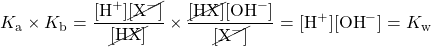Chapter 13. Chemical Equilibrium

# End-of-Chapter Material

1. What is the relationship between the Ksp expressions for a chemical reaction and its reverse chemical reaction?
2. What is the relationship between the Kw value for H2O and its reverse chemical reaction?
3. For the equilibrium

PCl3(g) + Cl2(g) ⇄ PCl5(g) + 60 kJ

list four stresses that serve to increase the amount of PCl5.

4. For the equilibrium

N2O4 + 57 kJ ⇄ 2NO2

list four stresses that serve to increase the amount of NO2.

5. Does a very large Keq favour the reactants or the products? Explain your answer.
6. Is the Keq for reactions that favour reactants large or small? Explain your answer.
7. Show that Ka × Kb = Kw by determining the expressions for these two reactions and multiplying them together.

HX(aq) ⇄ H+(aq) + X(aq)
X(aq) + H2O(ℓ) ⇄ HX(aq) + OH(aq)

8. Is the conjugate base of a strong acid weak or strong? Explain your answer.
9. What is the solubility in moles per litre of AgCl? Use data from Table 13.2 “Solubility Product Constants for Slightly Soluble Ionic Compounds”.
10. What is the solubility in moles per litre of Ca(OH)2? Use data from Table 13.2.
11. Under what conditions is Keq = KP?
12. Under what conditions is Keq > KP when the temperature is 298 K?
13. What is the pH of a saturated solution of Mg(OH)2? Use data from Table 13.2.
14. What are the pH and the pOH of a saturated solution of Fe(OH)3? The Ksp of Fe(OH)3 is 2.8 × 10−39.
15. For a salt that has the general formula MX, an ICE chart shows that the Ksp is equal to x2, where x is the concentration of the cation. What is the appropriate formula for the Ksp of a salt that has a general formula of MX2?
16. Referring to Exercise 15, what is the appropriate formula for the Ksp of a salt that has a general formula of M2X3 if the concentration of the cation is defined as 2x, rather than x?
17. Consider a saturated solution of PbBr2(s). If [Pb2+] is 1.33 × 10−5 M, find each of the following.
1. [Br−]
2. the Ksp of PbBr2(s)
18. Consider a saturated solution of Pb3(PO4)2(s). If [Pb2+] is 7.34 × 10−14 M, find each of the following.
1. [PO43−]
2. the Ksp of Pb3(PO4)2(s)

1. They are reciprocals of each other.
1. increase the pressure; decrease the temperature; add PCl3; add Cl2; remove PCl5
1. favour products because the numerator of the ratio for the Keq is larger than the denominator
1.1. 1.3 × 10−5 mol/L
1. Keq = KP when the number of moles of gas on both sides of the reaction is the same.
1. 10.35
1. 4x3
1. 2.66 × 10−5 M
2. 9.41 × 10−15